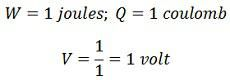# What is potential difference

Definition: The electrical potential is defined as the capability of the charged body to do work. When the body is charged, either electric electrons are supplied to it, or they are removed from it. In both the cases, the work is done. This work is stored in the body in the form of electric potential. Thus, the body can do the work by exerting a force of attraction or repulsion on the other charged particles.The capacity of the charged body to do work determines the electrical potential on it. The measure of the electrical potential is the work done to charge a body to one coulomb, i.e.,Units: Since the work done is measured in joules and charge in coulombs, the unit of electric potential is joules /coulombs, the unit of electric potential is joules/coulomb or volts.Hence a body is said to have an electrical potential of 1 volt if one joule of work is done to charge the body to one coulomb.

### Electric Potential Difference

The electrical potential difference is defined as the amount of work done to carrying a unit charge from one point to another in an electric field. In other words, the potential difference is defined as the difference in the electric potential of the two charged bodies.When a body is charged to a different electric potential as compared to the other charged body, the two bodies are said to a potential difference. Both the bodies are under stress and strain and try to attain minimum potential

Unit: The unit of potential difference is volt.